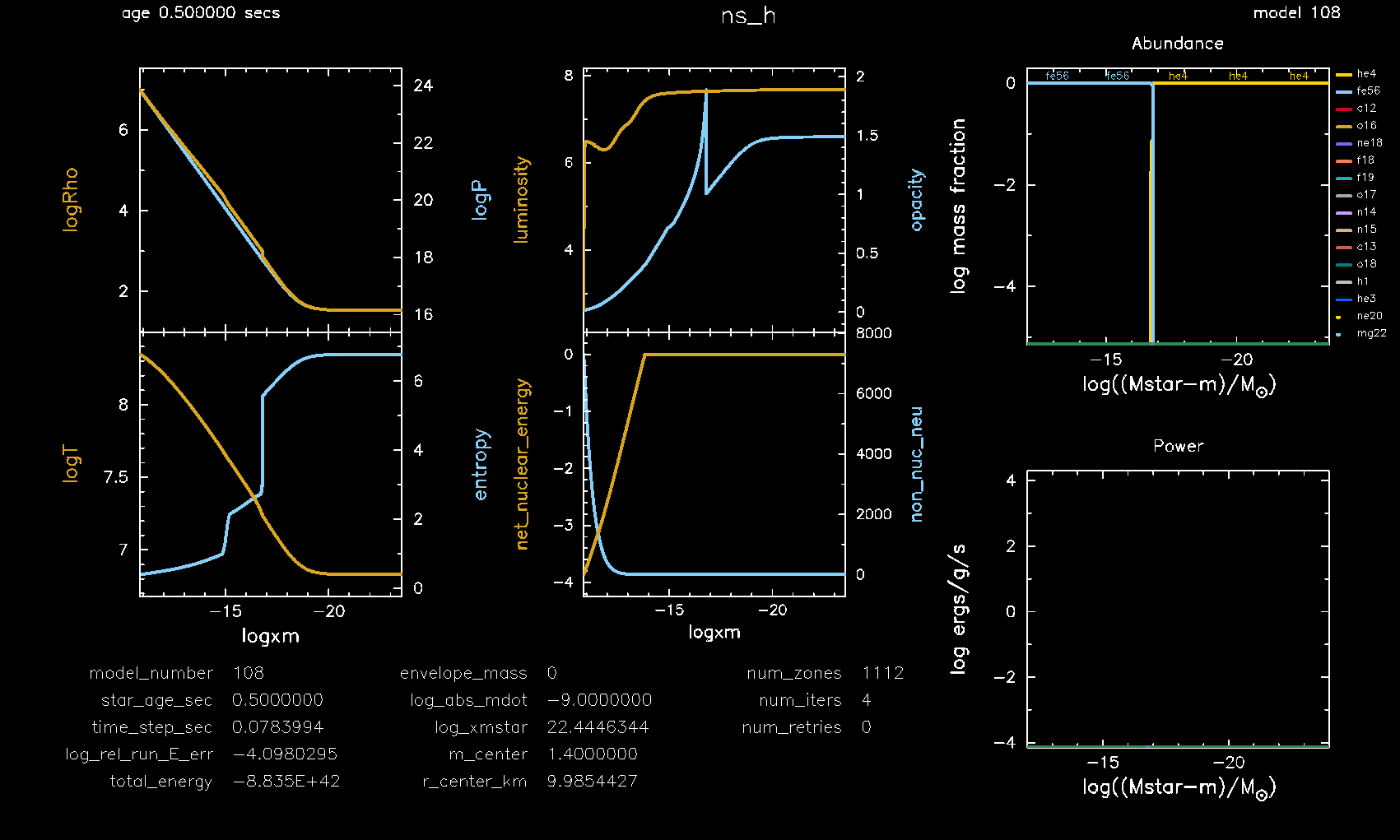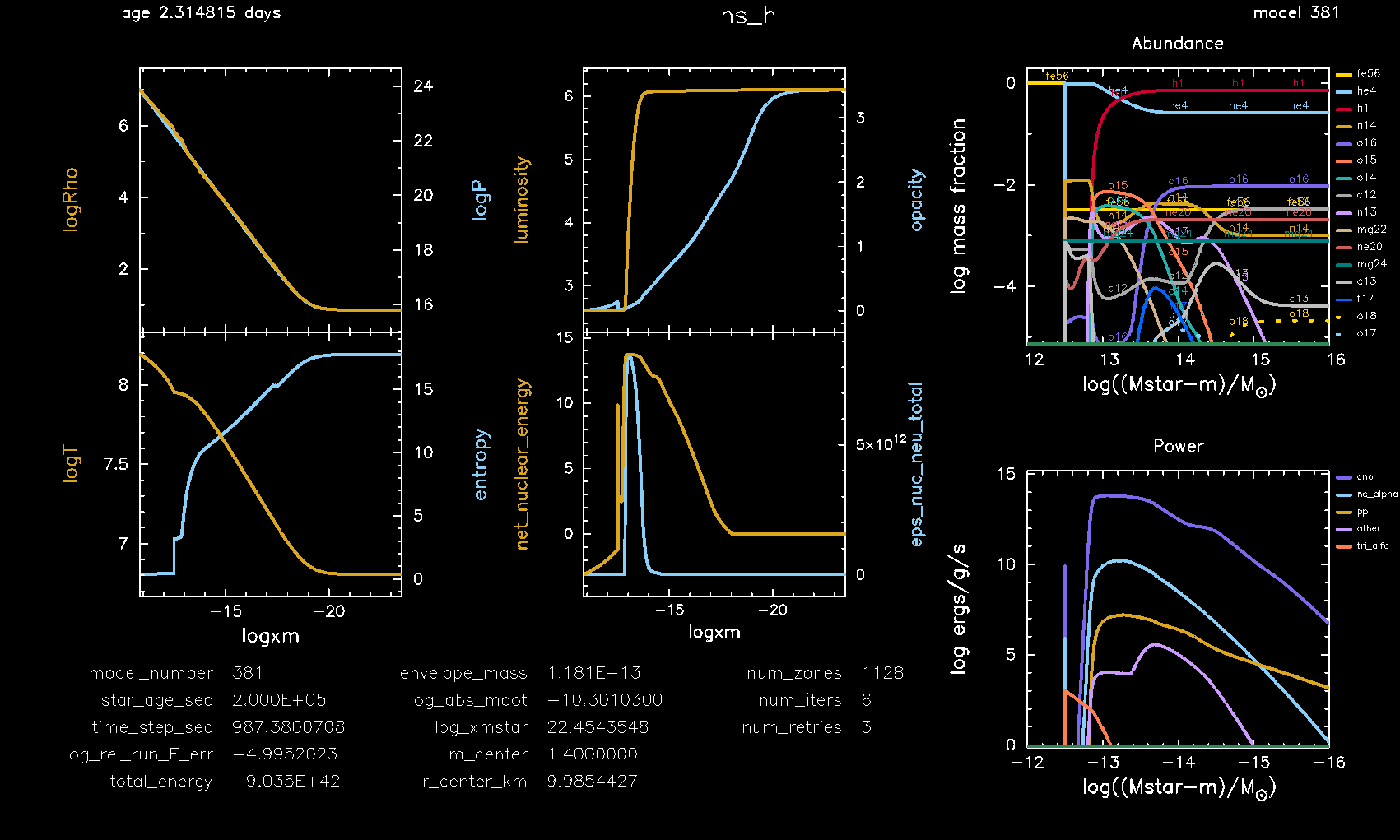# ns_h¶

This test case shows an example of steady hydrogen burning within a neutron star envelope.

This test case has 2 parts. Click to see a larger version of a plot.

• Part 1 (inlist_add_he_layer) first loads a prebuilt neutron star envelope model ns_env.mod, see the make_env test case. Helium is then accreted at a rate of 1e-9 Msun/year for 0.5 seconds to create a thin helium layer:• Part 2 (inlist_to_steady_h_burn) continues the evolution by accreting a hydrogen-rich solar mixture at a rate of 1e-11 Msun/year. Hydrogen burning eventually reaches a steady location, logxm = log10((Mstar - m) / Msun) = -12.8, within the neutron star envelope after 2e5 seconds of evolution:pgstar commands used for the plot above:

&pgstar

file_white_on_black_flag = .true. ! white_on_black flags -- true means white foreground color on black background
!file_device = 'png'            ! png
!file_extension = 'png'

file_device = 'vcps'          ! postscript
file_extension = 'ps'

pgstar_interval = 10

pgstar_grid_title_disp = 1.8

Abundance_xaxis_name = 'logxm'
Abundance_xaxis_reversed = .true.
Abundance_xmin = -16.0
Abundance_xmax = -12.0

Power_xaxis_name = 'logxm'
Power_xaxis_reversed = .true
Power_xmin = -16.0
Power_xmax = -12.0

Profile_Panels1_title = ''
Profile_Panels1_num_panels = 2

Profile_Panels1_xaxis_name = 'logxm'
Profile_Panels1_xaxis_reversed = .true.
Profile_Panels1_xmin = -101d0
Profile_Panels1_xmax = -101d0

Profile_Panels1_yaxis_name(1) = 'logRho'
Profile_Panels1_yaxis_name(2) = 'logT'
Profile_Panels1_ymin(1) = -101

Profile_Panels1_other_yaxis_name(1) = 'logP'
Profile_Panels1_other_yaxis_name(2) = 'entropy'
Profile_Panels1_other_ymin(1) = -101

Profile_Panels2_title = ''
Profile_Panels2_num_panels = 2

Profile_Panels2_xaxis_name = 'logxm'
Profile_Panels2_xaxis_reversed = .true.
Profile_Panels2_xmin = -101d0
Profile_Panels2_xmax = -101d0

Profile_Panels2_yaxis_name(1) = 'luminosity'
Profile_Panels2_yaxis_name(2) = 'net_nuclear_energy'
Profile_Panels2_ymin(1) = -101
Profile_Panels2_ymin(2) = -101

Profile_Panels2_other_yaxis_name(1) = 'opacity'
Profile_Panels2_other_yaxis_name(2) = 'eps_nuc_neu_total'
Profile_Panels2_other_ymin(1) = -101
Profile_Panels2_other_ymin(2) = -101

Text_Summary1_txt_scale = 5.5

Text_Summary1_num_rows = 5
Text_Summary1_num_cols = 3
Text_Summary1_name(1, 1) = 'model_number'
Text_Summary1_name(2, 1) = 'star_age_sec'
Text_Summary1_name(3, 1) = 'time_step_sec'
Text_Summary1_name(4, 1) = 'log_rel_run_E_err'
Text_Summary1_name(5, 1) = 'total_energy'
Text_Summary1_name(1, 2) = 'envelope_mass'
Text_Summary1_name(2, 2) = 'log_abs_mdot'
Text_Summary1_name(3, 2) = 'log_xmstar'
Text_Summary1_name(4, 2) = 'm_center'
Text_Summary1_name(5, 2) = 'r_center_km'
Text_Summary1_name(1, 3) = 'num_zones'
Text_Summary1_name(2, 3) = 'num_iters'
Text_Summary1_name(3, 3) = 'num_retries'
Text_Summary1_name(4, 3) = ' '
Text_Summary1_name(5, 3) = ' '

Grid2_title = 'ns_h'
Grid2_plot_name(1) = 'Profile_Panels1'
Grid2_plot_name(2) = 'Text_Summary1'
Grid2_plot_name(3) = 'Abundance'
Grid2_plot_name(4) = 'Power'
Grid2_plot_name(5) = 'Profile_Panels2'
Grid2_plot_row(1) = 1
Grid2_plot_row(2) = 7
Grid2_plot_row(3) = 1
Grid2_plot_row(4) = 5
Grid2_plot_row(5) = 1
Grid2_plot_rowspan(1) = 6
Grid2_plot_rowspan(2) = 2
Grid2_plot_rowspan(3) = 4
Grid2_plot_rowspan(4) = 4
Grid2_plot_rowspan(5) = 6
Grid2_plot_col(1) = 1
Grid2_plot_col(2) = 1
Grid2_plot_col(3) = 5
Grid2_plot_col(4) = 5
Grid2_plot_col(5) = 3
Grid2_plot_colspan(1) = 2
Grid2_plot_colspan(2) = 4
Grid2_plot_colspan(3) = 3
Grid2_plot_colspan(4) = 3
Grid2_plot_colspan(5) = 2
Grid2_txt_scale_factor(1) = 0.65
Grid2_txt_scale_factor(2) = 0.19
Grid2_txt_scale_factor(3) = 0.65
Grid2_txt_scale_factor(4) = 0.65
Grid2_txt_scale_factor(5) = 0.65

Grid2_num_cols = 7
Grid2_num_rows = 8
Grid2_num_plots = 5

Grid2_win_flag = .true.
Grid2_win_width = 16
Grid2_win_aspect_ratio = 0.6

Grid2_file_flag = .true.
Grid2_file_dir = 'pgstar_out'
Grid2_file_prefix = 'grid_'
Grid2_file_interval = 10000
Grid2_file_width = 20
Grid2_file_aspect_ratio = -1

/ ! end of pgstar namelist


Last-Updated: 21Jun2021 (MESA e2acbc2) by fxt.